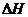Chapter 10, Problem 48QAP### Introductory Chemistry: A Foundati...

9th Edition
Steven S. Zumdahl + 1 other
ISBN: 9781337399425

#### Solutions

Chapter
Section### Introductory Chemistry: A Foundati...

9th Edition
Steven S. Zumdahl + 1 other
ISBN: 9781337399425
Textbook Problem
1 views

# Given the following data:mg src=Images/HTML_99425-10-48QAP_image001.jpg alt="" align="top"/>lculatefor the reactionmg src=Images/HTML_99425-10-48QAP_image003.jpg alt="" align="top"/>

Interpretation Introduction

Interpretation:

The enthalpy change for the following reaction should be determined.

2C(s)+H2(g)C2H2(g)

Concept Introduction:

Enthalpy of a reaction is a state function thus; change in enthalpy of a reaction does not depend on the path of the reaction.

The change in enthalpy is same if the same reaction takes place in one step or series of steps. This is known as Hess’s law. There are following characteristics of change in enthalpy which are important to calculate the change in enthalpy of a reaction using Hess’s law.

1. For a reversed reaction, the sign of enthalpy changes of the reaction also reversed.
2. If any reactant or product is multiplied by any integer in the chemical reaction, enthalpy change of that reactant or product is also multiplied by the same integer.
Explanation

Given Information:

The following reactions are given:

C2H2(g)+52O22CO2(g)+H2O(l)   ΔH=1300 kJC(s)+O2(g)CO2(g)                        ΔH=394 kJH2(g)+12O2(g)H2O(l)                   ΔH=286 kJ

Calculation:

The net reaction is as follows:

2C(s)+H2(g)C2H2(g)

To obtain the above reaction,

Multiply the 2nd reaction by 2 thus,

2C(s)+2O2(g)2CO2(g)                        ΔH=788 kJ

Reverse the 1st reaction as follows:

2CO2(

### Still sussing out bartleby?

Check out a sample textbook solution.

See a sample solution

#### The Solution to Your Study Problems

Bartleby provides explanations to thousands of textbook problems written by our experts, many with advanced degrees!

Get Started